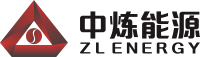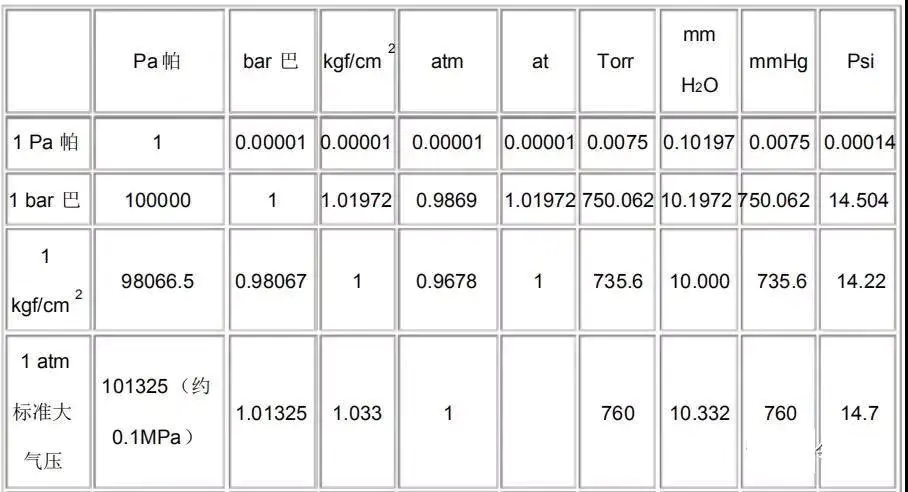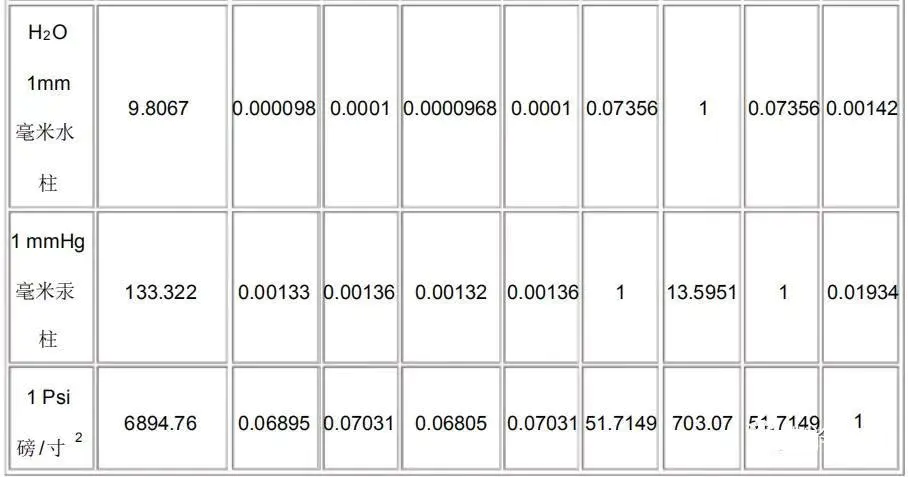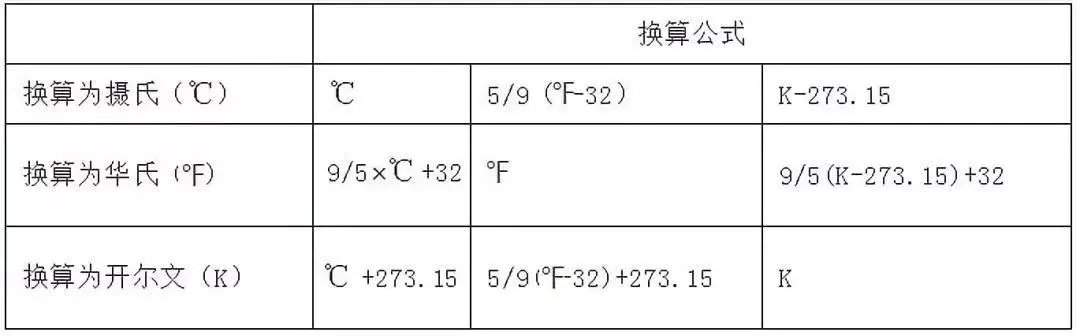1 MW（兆瓦）=1000 kW（千瓦）

1 kW=1000 W

1 kW=861 kcal/h（大卡）=0.39 P（冷量）

1 W= 1 J/s（焦/秒）

0.1 MPa=1kg/cm2=10 mH2O=100 kPa

1 USRT（美国冷吨）=3024 kcal/h=3517 W（冷量）

1 BTU（英热单位）=0.252kcal/h=1055 J

1 BTU/h（英热单位/小时）=0.252 kcal/h

1 BTU/h（英热单位/小时）=0.2931 W（冷量）

1 MTU/h（千英热单位/小时）=0.2931 kW（冷量）

1 HP（电）=0.75 kW（电）

1 kW（电）=1.34 HP（电）

1 RT(（冷量）=3.517 kW（冷量）

1 kW（冷量）=0.284 RT=860 kcal/h=3.412 MBH（103英热单位/小时）

1 P（冷量）=2200 kcal/h=2.56 kW

1 kcal/h=1.163 W

1 W=0.86 kcal/h

℉（华氏度）=9/5℃+32

℃（摄氏度）=（℉-32）5/9

1 CFM（立方英尺/分钟）=1.699 m³/h=0.4719 L/s

1 m³/h=0.5886 CFM（立方英尺/分钟）

1 L/s=2.119 CFM（立方英尺/分钟）

1 GPM（加仑/分钟）=0.06308 L/s

1 L/s=15.85 GPM（加仑/分钟）

1 kg/cm2=10 mH2O=1 bar=0.1 MPa

1 Pa=0.1 mmH2O=0.0001 mH2O

1 mH2O=104 Pa=10 kPa

1kcal（大卡）：标准大气压下1公斤水温度上升或下降1℃，所吸收或者释放的热量就是1大卡。

EER：在夏季制冷时，制冷量（W或Btu/h）与输入功率（W）的比率定义为热泵的能效比。

COP：在冬季供热时，制热量（W）与输入功率（W）的比率定义为热泵的循环性能系数。

IPLV：综合部分负荷性能系数 是用一个单一数值表示空气调节用冷水机组的部分负荷效率指标。